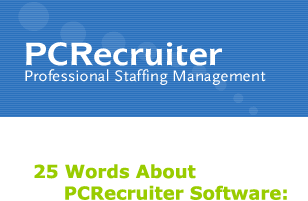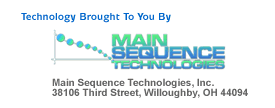William Kubicek on Sun, 10 Nov 2002 17:51:10 +0100 (CET)

Title: PCRecruiter
=0d=0a=0d=0a

=0d=0a

=0d=0a=0d=0a=0d=0a=0d=0a=0d=0a=0d=0a=0d=0a=0d=0a=0d=0a=0d=0a=0d=0a =0d=0a=0d=0aInexpensive Microsoft technology =0d=0aWorks with Outlook, Lotus Notes, Excel, Access/SQL, Word =0d=0aUsed by over 1500 organizations =0d=0aAvailable as installed Windows or Web software, or ASP =0d=0a=0d=0a
=0d=0a=0d=0a=0d=0a=0d=0a=0d=0a=0d=0a=0d=0a=0d=0a=0d=0a=0d=0a=0d=0a=0d=0aPCRecruiter empowers you with the ability to:

> Send bulk emails
> Create customized candidate profiles
> Create call lists
> Export to Excel
> Combine databases
> Print reports (EEO)
> Merge records
> And a host of other tasks
=0d=0a
=0d=0a=0d=0a=0d=0a=0d=0a=0d=0a
 Call (440) 946-5214 today for a risk-free, no-cost demo!
=0d=0a
=0d=0a=0d=0a=0d=0a=0d=0a=0d=0a=0d=0a

If you have received this email in error you may unsubscribe by clicking here=2e

This document is provided for information only=2e This information is not a promise or guarantee nor is the accuracy of any information generated after this item is published guaranteed=2e This document is provided AS IS without warranty of any kind, express or implied, including but not limited to merchant=2e